##### Quantum Physics For Dummies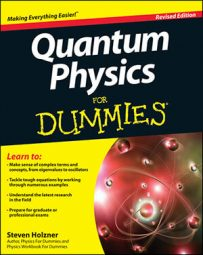If your quantum physics instructor asks you to solve for the wave function of the center of mass of the electron/proton system in a hydrogen atom, you can do so using a modified Schrödinger equation: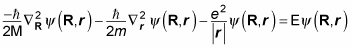What you will find is that you can actually ignore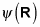and go straight on to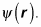Here’s how it works.

Because the Schrödinger equation contains terms involving either R or r but not both, the form of this equation indicates that it’s a separable differential equation. And that means you can look for a solution of the following form: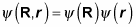Substituting the preceding equation into the one before it gives you the following: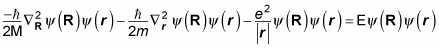And dividing this equation by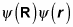gives you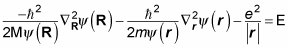This equation has terms that depend on either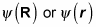but not both. That means you can separate this equation into two equations, like this (where the total energy, E, equals ER + Er):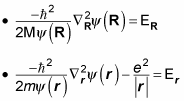Multiplying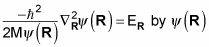gives you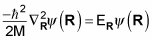And multiplying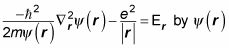gives you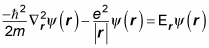Now you have two Schrödinger equations, which you can solve independently.

So, using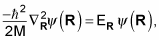you can now solve for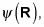which is the wave function of the center of mass of the electron/proton system. This is a straightforward differential equation, and the solution is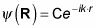Here, C is a constant and k is the wave vector, where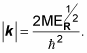In practice, however, ER is so small that people almost always just ignore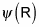— that is, they assume it to be 1. In other words, the real action is in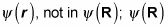is the wave function for the center of mass of the hydrogen atom, and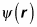is the wave function for a (fictitious) particle of mass m.